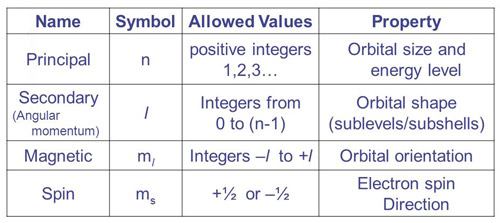A# quantum numberQuantum numbers: summary.

A quantum number is any of four numbers used to classify electrons and their atomic states. In particular, these numbers characterize the electron configuration of an atom. The four quantum numbers are: the principal quantum number, the azimuthal quantum number, the magnetic quantum number, and the spin quantum number.

## Principal quantum number

The principal quantum number, n (= 1, 2, 3, ...) is used to label electron shells, i.e., to denote the overall size and energy of an electron shell. n may take on integer values from 1 to infinity.

## Azimuthal quantum number

The azimuthal quantum number, l (= 0, 1, 2, ..., n – 1), denotes the angular momentum and shape of an orbital (i.e., the most probable electron distribution). Azimuthal quantum numbers 0, 1, 2, and 3 correspond with the s, p, d, and f orbitals, respectively.

## Magnetic quantum number

The magnetic quantum number, ml (= –l, –l + 1, ... 0, ... l – 1, l), denotes the orientation of an orbital and labels different orbitals within a subshell. The number of orbitals in a subshell is the same as the number of possible m values.

## Spin quantum number

The spin quantum number, ms (= ± ½).